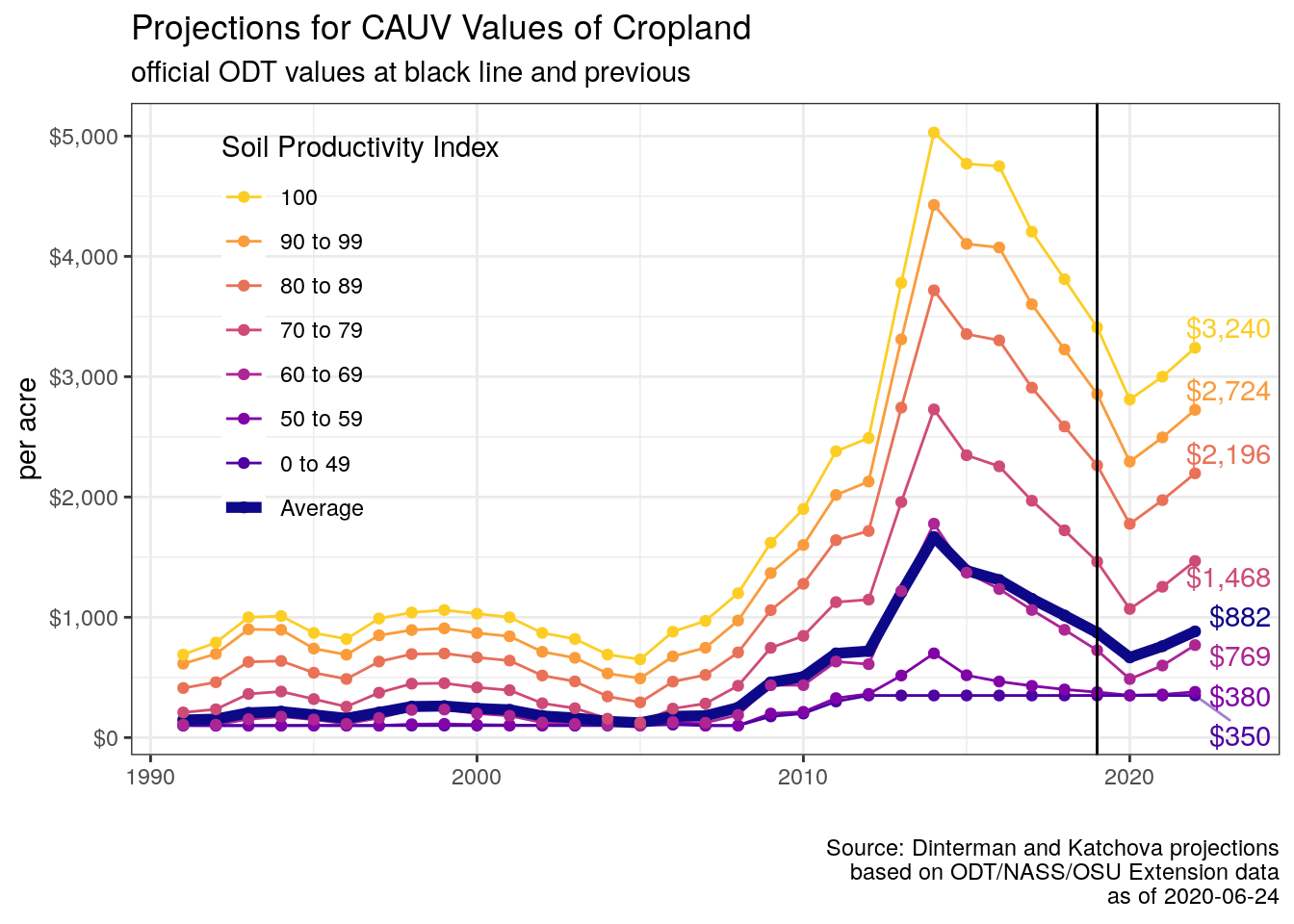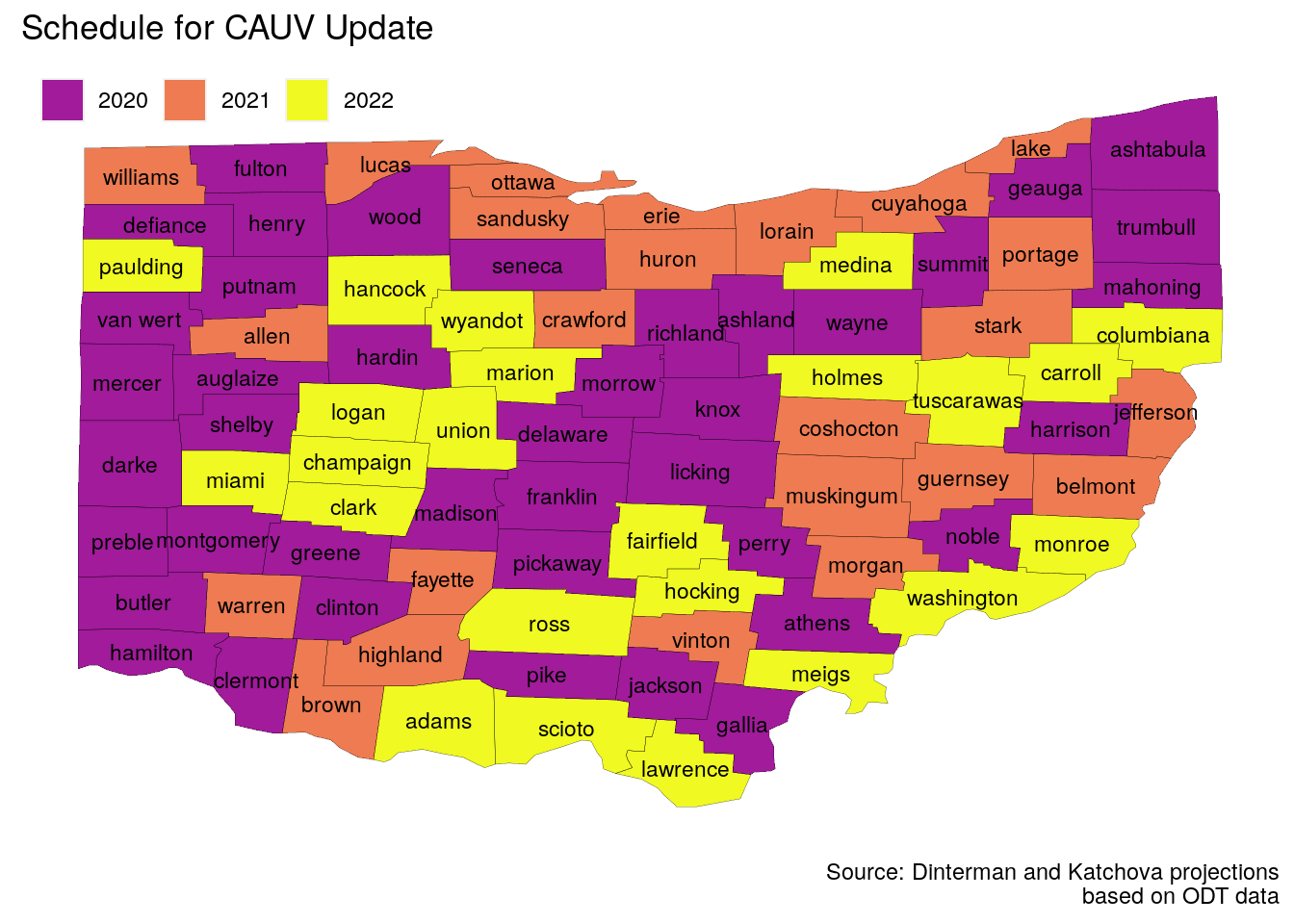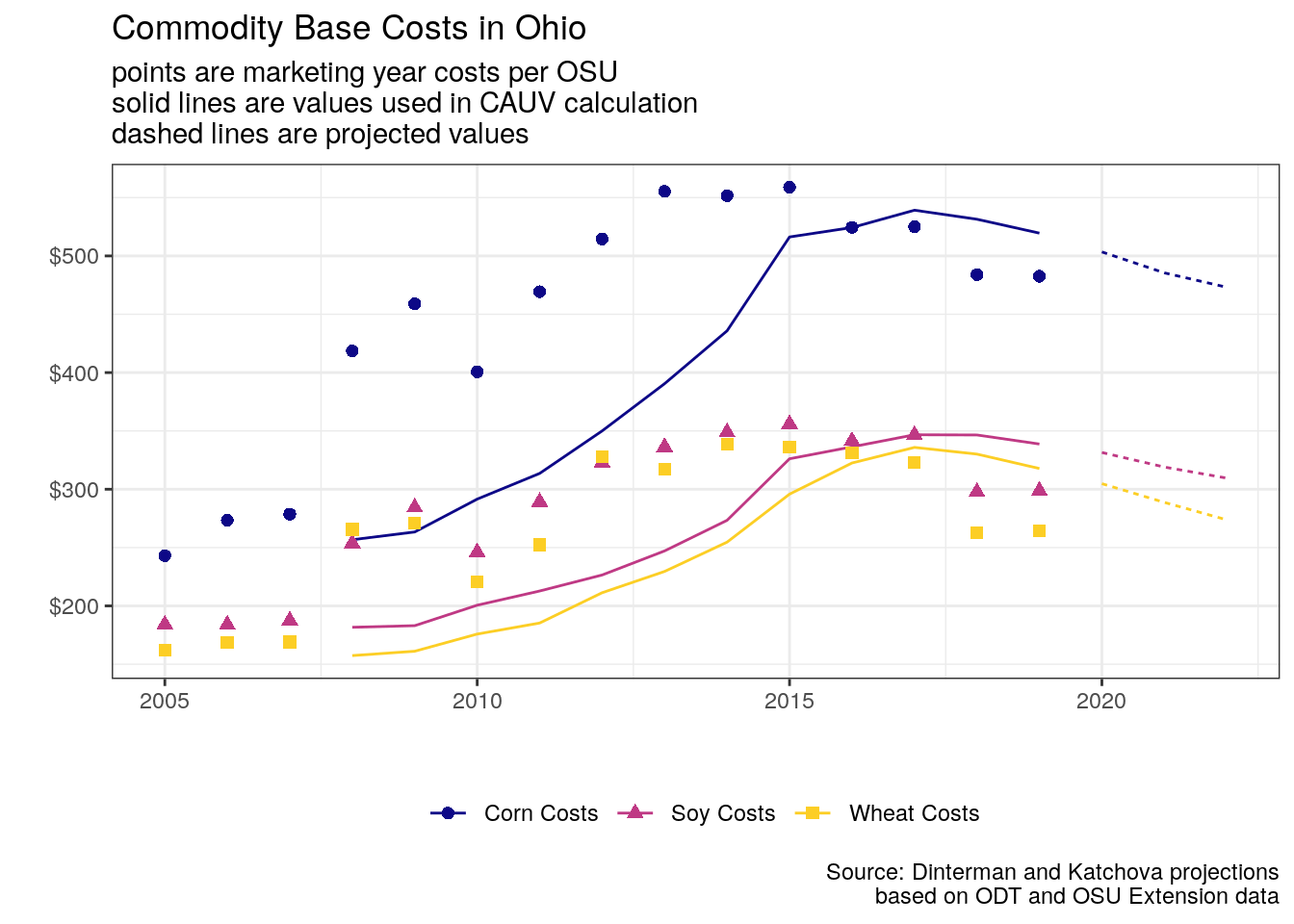# Key Findings

## Highlights

• Current projection for average CAUV value in 2020 is $665 which would represent a change of -24% from the previous tax year. This is the first year without the “phase-in”. • From 2017-2020 a -42% decrease in average CAUV values for counties receiving updates to their property taxes. • The projection for the average CAUV value in 2021 is$760. The average CAUV value projections could end up as low as $560 or as high as$900.
• From 2018-2021 a -25% change from the previous triennial update.
• The very early projection for the average CAUV value in 2022 is $880. The average CAUV value projections could end up as low as$665 or as high as $1,010. • From 2019-2022 a 1% change from the previous triennial update. ## Projected CAUV Expectations • Non-land costs have trended downward since 2015 and since this component is based off of an Olympic Average of the past 7 years, high values for non-land costs will be dropping out and this component is expected to continue to decrease. • Prices for corn, soybeans, and wheat have stagnated and are projected at current levels. Component based off of an Olympic Average of the past 8 years (excludes current). High values from 2012 and 2013 are dropping out. • Yields are based off of the past 11 years (excludes current) and have mostly continued along the path of a 30-year trendline upward. • Capitalization rate is based on an Olympic Average of the past 7 years which has recently been trending downward. Current Federal Reserve policy is pushing downward pressure on capitalization rate into the future. ## Expectations## Expectations Expected CAUV Projections Year avg_cauv indx_49 indx_59 indx_69 indx_79 indx_89 indx_99 indx_100 2007$181 $100$100 $123$283 $521$747 $970 2008$249 $100$100 $188$431 $708$973 $1,200 2009$459 $176$200 $435$746 $1,059$1,368 $1,620 2010$505 $200$214 $436$845 $1,278$1,601 $1,900 2011$700 $300$328 $632$1,126 $1,641$2,017 $2,380 2012$719 $350$363 $610$1,147 $1,717$2,128 $2,490 2013$1,205 $350$516 $1,218$1,958 $2,743$3,310 $3,780 2014$1,668 $350$700 $1,778$2,728 $3,718$4,428 $5,030 2015$1,388 $350$518 $1,371$2,347 $3,354$4,104 $4,770 2016$1,310 $350$466 $1,235$2,255 $3,302$4,074 $4,750 2017$1,153 $350$430 $1,061$1,969 $2,909$3,602 $4,205 2018$1,015 $350$400 $896$1,723 $2,586$3,226 $3,810 2019$873 $350$378 $725$1,462 $2,263$2,855 $3,410 2020$666 $350$351 $487$1,069 $1,777$2,294 $2,810 2021$759 $350$359 $599$1,254 $1,974$2,495 $3,000 2022$882 $350$380 $769$1,468 $2,196$2,724 \$3,240# CAUV Calculation

## CAUV Calculation Overview

It is the expected net present value of an acre of land based. To determine this, first a historical average of yields and prices for corn, soybeans, and wheat to determine gross income. Then historical non-land costs – compliments of The Ohio State University Agricultural Extension Agency – are subtracted from gross income for a measure of net income. And finally, this net income is divided by a capitalization rate based upon historical values of farm interest and equity rates. This value will vary based upon the particular soil type(s) for a farm.

## CAUV Calculation Overview

• A CAUV value is assigned for each of the 3,514 soil types in Ohio
• based on their expected yields of corn, soybeans, and wheat from the last state-wide soil survey in 1984
• Value is the expected net present value based on the past 5 to 10 years worth of data for each component
• Economics jargon, it is the net operating income divided by capitalization rate
• Net operating income is based off of the expected revenue (price times yield) less the expected costs (only non-land costs). Calculated for corn, soybeans, and wheat.
• Capitalization rate is a combination of the interest rate on a loan for purchasing farmland along with appreciation rate of farmland.

## Generic Formula

• CAUV is assigned for each soil type, although what really matters is the yields that soil type had for corn, soybeans, and wheat in 1984:

$CAUV_{s,t} = \frac{NOI_{s,t}}{CAP_t}$

• where $$CAP_t$$ represents the capitalization rate and $$NOI_{s,t}$$ represents the net operating income based on revenues less non-land costs for corn, soybeans, and wheat.
• Net operating income, $${NOI_{s,t}}$$, captures the average returns to an acre of land under normal management practices which is adjusted by the state-wide rotation pattern of commodities.

## Generic Formula

• $${NOI_{s,t}}$$ can be defined as:

$NOI_{s,t} = \sum_{c} w_{c,t}\times(GOI_{s,c,t} - {nonland}_{s,c,t})$

• where $$w_{c,t}$$ represents a commodities share of total harvested acreage
• $$GOI_{s,c,t}$$ is the expected revenues for a soil type depending on the statewide price of a commodity and yield is a percentage adjustment to the soil’s yield in 1984 for each commodity:

$GOI_{s,c,t} = Price_{c,Ohio,t} \times \left( \frac{Yield_{c,Ohio,t}}{Yield_{c,Ohio,1984}} \times Yield_{c,s,1984} \right)$

## Non-Land Costs